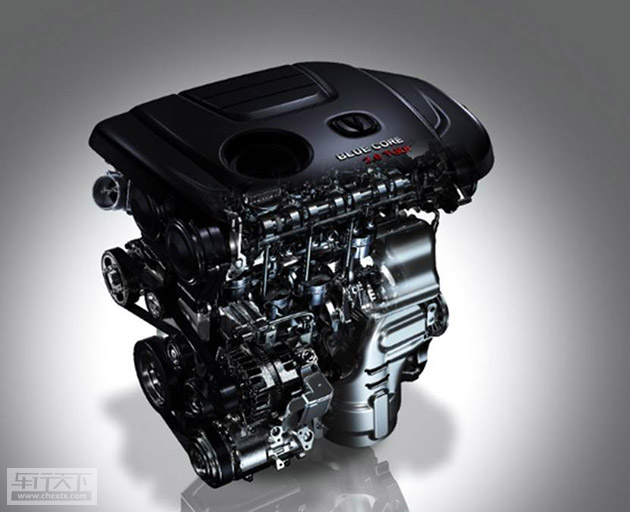## 馬力和速度來證明品質 長安CS95完勝秘籍

2017年01月10日 16:04 來源：www.fusbci.live 超過：83211次關注

夢想有一躍而起的驚喜，夢想擁有說走就走旅行，座駕的動力配備是實現夢想的前提條件。作為長安汽車品牌CS家族中最完美的SUV,長安CS95車型一經亮相，便成經典。

除了其來自意大利時尚設計之都——都靈設計中心別具一格的硬朗外型設計以外，英國伯明翰動力研發中心和美國底特律底盤研發中心的頂尖全球智慧，協同博世、博格華納、愛信等全球頂級的供應商伙伴，歷時6年，2000多個晝夜，傾力打造了這款“高性能全尺寸旗艦SUV”。強勁動力是高性能的外在體現，長安CS95的動力組成，是最為吸引人的亮點，是成就品質座駕的最強三劍客。

長安CS95擁有最強“中國芯”的藍鯨2.0TGDI發動機，為長安汽車自主研發，應用TC、GDI、DVVT、電子節溫器、電磁閥控制二級變排量機油泵、寬域氧傳感器、空氣質量流量計、平衡軸、OAD皮帶輪和帶LIN調節器的智能發電機等先進技術，全面提升發動機動力性、燃油經濟性、NVH和排放性能。

藍鯨2.0TGDI發動機作為CS95車型的心臟，成功的運用了新技術——TC-廢氣渦輪增壓技術，致使功率提升50%以上、中低速扭矩提升50%以上、整車油耗降低10-15%、是降低排放水平的關鍵；并顯著提升駕駛推背感，發動機在1500rmp即可明顯感覺到扭矩迅速提升，相比同級車型扭矩提升更快。

而在提高整車舒適性及發動機的可靠性方面，藍鯨D20TGDI發動機應用的平衡軸技術，可最大限度的降低發動機運行不平衡的因素，改善一般的直列四缸發動機，在運行時，二階往復慣性力不平衡導致發動機振動大為駕乘人員帶來的不舒適性。

長安CS95車型的最大功率171Kw，在1750rpm-3500rpm之間持續爆發360N·m的最大扭矩，已經達到了與國際品牌大型SUV發動機的同級水平。發動機一經啟動，猶如一條藍鯨一擺尾鰭，驅動身體充滿力量的向前行進，彰顯了瞬間爆發力和強大的力量感。如此強大的發動機設備，瞬間秒殺了大部分主流合資車型。長安CS95所搭載的愛信TF-81SC 6AT變速箱，最大可承載450N·m的扭矩，具有傳遞效率高效、燃油經濟性、控制功能豐富等特點，快速換擋可以在0.8秒之內實現響應。就如同藍鯨2.0TGDI發動機強大的消化系統，能夠迅速消化動力能量，提升加速度。讓藍鯨猶如在浩瀚大海中，吃飽之后全力而行，迅猛而靈敏。長安CS95的四驅車型所采用的國際領先的博格華納全新一代NexTrac 智能四驅電子控制系統，具備強大的越野持續脫困能力。無論是蜿蜒盤行的山間，還是溝坎從生的險路，都能夠如履平地。它可以根據道路狀況合理的分配前后輪扭矩，同時為車輛帶來更高效的驅動性能和起步加速性能。如藍鯨的左膀右臂般，配合整個動力系統，支持龐大的身軀暢行無阻。“藍鯨2.0TGDI發動機+愛信TF-81SC 6AT變速箱+博格華納NexTrac四驅系統”，就是長安CS95車型的動力三劍客，是整車高性能的核心力量，是提供前行的重要動力。試想一下，當您駕駛著長安CS95車型，強大的動力系統不僅為您節省了行駛時間，還為您節約了不少的自駕成本。擁有這樣的一款座駕，能夠讓您體驗到高性能動力系統所帶來的駕乘舒適感和對速度的追求。

作為中國汽車品牌的扛鼎之作，長安CS95以強勁的動力和速度展現了高品質汽車的高性能特點，用自主研發的一流尖端技術完勝了主流合資品牌車型，填補國內2.0L及以上增壓直噴發動機自主開發能力空白，用國內最強，世界領先的發動機系統，證明了中國力量。

#### 相關文章

0-500 字已有評論 0條 查看評論>>

### 熱門標簽

?
• 快速找車
• 選擇品牌
• 選擇品牌
• A  奧迪
• A  阿斯頓·馬丁
• A  阿爾法·羅密歐
• B  寶沃
• B  布加迪
• B  巴博斯
• B  保時捷
• B  賓利
• B  奔馳
• B  寶馬
• B  本田
• B  別克
• B  標致
• B  比亞迪
• B  寶駿
• B  北汽制造
• B  北汽新能源
• B  北汽幻速
• B  北汽威旺
• B  北京汽車
• B  奔騰
• B  北汽紳寶
• C  長安
• C  長安商用
• C  長城
• C  昌河
• D  大眾
• D  道奇
• D  DS
• D  東南
• D  東風風神
• D  東風風行
• D  東風小康
• D  東風風度
• D  東風
• F  福特
• F  豐田
• F  菲亞特
• F  法拉利
• F  福田
• F  福迪
• F  福汽啟騰
• G  觀致
• G  廣汽傳祺
• G  廣汽吉奧
• G  GMC
• H  紅旗
• H  漢騰汽車
• H  哈弗
• H  哈飛
• H  海格
• H  海馬
• H  華頌
• H  黃海
• H  華泰
• H  恒天
• J  吉利汽車
• J  捷豹
• J  Jeep
• J  江淮
• J  江鈴
• J  金杯
• J  九龍
• J  金旅
• K  凱翼
• K  凱迪拉克
• K  克萊斯勒
• K  科尼塞克
• K  卡威
• K  開瑞
• L  路虎
• L  林肯
• L  勞斯萊斯
• L  蘭博基尼
• L  雷克薩斯
• L  鈴木
• L  雷諾
• L  理念
• L  力帆
• L  蓮花汽車
• L  獵豹
• L  路特斯
• L  陸風
• M  馬自達
• M  MG
• M  MINI
• M  瑪莎拉蒂
• M  摩根
• M  邁凱輪
• N  納智捷
• O  歐寶
• O  謳歌
• O  歐朗
• Q  奇瑞
• Q  起亞
• Q  啟辰
• R  日產
• R  榮威
• R  瑞麒
• S  三菱
• S  斯威汽車
• S  薩博
• S  smart
• S  斯柯達
• S  斯巴魯
• S  思銘
• S  雙龍
• S  上汽大通
• S  雙環
• T  特斯拉
• T  騰勢
• W  沃爾沃
• W  五菱汽車
• W  五十鈴
• W  威茲曼
• W  威麟
• X  現代
• X  雪佛蘭
• X  雪鐵龍
• X  西雅特
• Y  一汽
• Y  英菲尼迪
• Y  英致
• Y  依維柯
• Y  野馬汽車
• Y  永源
• Z  眾泰
• Z  中華
• Z  中興
• Z  知豆
• 選擇車系
• 選擇車系
• 車型對比
• 選擇品牌
• 選擇品牌
• A  奧迪
• A  阿斯頓·馬丁
• A  阿爾法·羅密歐
• B  寶沃
• B  布加迪
• B  巴博斯
• B  保時捷
• B  賓利
• B  奔馳
• B  寶馬
• B  本田
• B  別克
• B  標致
• B  比亞迪
• B  寶駿
• B  北汽制造
• B  北汽新能源
• B  北汽幻速
• B  北汽威旺
• B  北京汽車
• B  奔騰
• B  北汽紳寶
• C  長安
• C  長安商用
• C  長城
• C  昌河
• D  大眾
• D  道奇
• D  DS
• D  東南
• D  東風風神
• D  東風風行
• D  東風小康
• D  東風風度
• D  東風
• F  福特
• F  豐田
• F  菲亞特
• F  法拉利
• F  福田
• F  福迪
• F  福汽啟騰
• G  觀致
• G  廣汽傳祺
• G  廣汽吉奧
• G  GMC
• H  紅旗
• H  漢騰汽車
• H  哈弗
• H  哈飛
• H  海格
• H  海馬
• H  華頌
• H  黃海
• H  華泰
• H  恒天
• J  吉利汽車
• J  捷豹
• J  Jeep
• J  江淮
• J  江鈴
• J  金杯
• J  九龍
• J  金旅
• K  凱翼
• K  凱迪拉克
• K  克萊斯勒
• K  科尼塞克
• K  卡威
• K  開瑞
• L  路虎
• L  林肯
• L  勞斯萊斯
• L  蘭博基尼
• L  雷克薩斯
• L  鈴木
• L  雷諾
• L  理念
• L  力帆
• L  蓮花汽車
• L  獵豹
• L  路特斯
• L  陸風
• M  馬自達
• M  MG
• M  MINI
• M  瑪莎拉蒂
• M  摩根
• M  邁凱輪
• N  納智捷
• O  歐寶
• O  謳歌
• O  歐朗
• Q  奇瑞
• Q  起亞
• Q  啟辰
• R  日產
• R  榮威
• R  瑞麒
• S  三菱
• S  斯威汽車
• S  薩博
• S  smart
• S  斯柯達
• S  斯巴魯
• S  思銘
• S  雙龍
• S  上汽大通
• S  雙環
• T  特斯拉
• T  騰勢
• W  沃爾沃
• W  五菱汽車
• W  五十鈴
• W  威茲曼
• W  威麟
• X  現代
• X  雪佛蘭
• X  雪鐵龍
• X  西雅特
• Y  一汽
• Y  英菲尼迪
• Y  英致
• Y  依維柯
• Y  野馬汽車
• Y  永源
• Z  眾泰
• Z  中華
• Z  中興
• Z  知豆
• 選擇車系
• 選擇車系
• 選擇車型
• 選擇車型
• 意見反饋